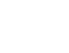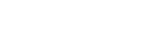# Financial Analytics – Part 3

### Introduction

Hi MLEnthusiasts! Here’s the part 3 of our financial analytics series. Today, we will be learning how to calculate the cumulative return of the portfolio of securities, given the returns of individual stocks, using python.

### The Theory

Before jumping on code, let’s first understand the theory behind calculating the cumulative return of portfolio of securities.

To calculate this, we need to know the expected returns of the individual assets and also the weight associated with each asset.

We get the cumulative return by multiplying the return of each individual asset with its associated weight and then summing these up. Thus, the cumulative return is the weighted average of individual returns.

Suppose, we have three assets A1, A2 and A3. The weights associated with these assets are w1, w2 and w3. Also, the returns associated with these assets are r1, r2 and r3. Let the cumulative return be represented as R. Thus, R is given by

R = w1r1+w2r2+w3r3

If there are N assets, the same R is given by

R = Σwnrn

where n varies from 1 to N.

### The Code

Okay, enough of the theory! Let’s now jump on doing this using python!

The first step is to import the libraries.

In :
```#Numpy is the package for scientific computing with python. Array computations are made very easy by using this package.
import numpy as np

#For data manipulation and data analysis. Manipulation of dataframes is made very easy using this library.
import pandas as pd

#Functions from pandas_datareader.data and pandas_datareader.wb extract data from various Internet sources into a pandas DataFrame.
from pandas_datareader import data as dr

#Using pyplot, we can get interactive plots and generate programmatic plots
import matplotlib.pyplot as plt
```

Next, we will make a list of assets – ‘PG'(Procter and Gamble), ‘MSFT'(Microsoft) and ‘GE'(General Electric), save it in a variable ‘assets’. Make a new blank dataframe, get it saved in assetsAdjClose and then bring the ‘Adj Close’ column of all these assets in this dataframe. We will fetch data from yahoo finance.

In :
```assets = ['PG', 'MSFT', 'GE']
for a in assets:
```
In :
```assetsAdjClose.info()     #Checking what's there in this dataframe!
```
```<class 'pandas.core.frame.DataFrame'>
DatetimeIndex: 6231 entries, 1995-01-03 to 2019-10-01
Data columns (total 3 columns):
PG      6231 non-null float64
MSFT    6231 non-null float64
GE      6231 non-null float64
dtypes: float64(3)
memory usage: 194.7 KB
```
In :
```assetsAdjClose.head()    #Checking first 5 rows of this dataframe
```
Out:
PG MSFT GE
Date
1995-01-03 8.472425 2.428365 4.082655
1995-01-04 8.404510 2.446017 4.082655
1995-01-05 8.285657 2.405671 4.092661
1995-01-06 8.302632 2.446017 4.072646
1995-01-09 8.268674 2.430887 4.032622
In :
```assetsAdjClose.tail()     #Checking last 5 rows of this dataframe
```
Out:
PG MSFT GE
Date
2019-09-25 123.019997 139.360001 9.16
2019-09-26 124.309998 139.539993 9.02
2019-09-27 124.570000 137.729996 9.04
2019-09-30 124.379997 139.029999 8.94
2019-10-01 123.849998 137.070007 8.61

## Normalization to 100:¶

We do normalization to keep the base of all the assets the same. This helps us in comparison. Here,
Po is the first row (data corresponding to the oldest date) of the dataframe and  Pis the next row.In :
```assetsAdjClose.iloc       #Gives the first row figures of the dataframe.
```
Out:
```PG      8.472425
MSFT    2.428365
GE      4.082655
Name: 1995-01-03 00:00:00, dtype: float64```
In :
```(assetsAdjClose/assetsAdjClose.iloc*100).plot(figsize = (15, 6)); #Normalizing the dataframe
plt.show()  #Plot the line chart of all the securities - data is normalized.
```
In :
```assetsAdjClose.plot(figsize=(15,6))
plt.show()     #line chart of data without normalization
```

## Calculating the Return of a Portfolio of Securities¶In :
```#pandas.Dataframe.shift(# lags)
#Using shift(1), we can get the row just above the present row. Here, # lags is 1.
returns.head()       #displaying first 5 rows of returns dataframe
```
Out:
PG MSFT GE
Date
1995-01-03 NaN NaN NaN
1995-01-04 -0.008016 0.007269 0.000000
1995-01-05 -0.014142 -0.016495 0.002451
1995-01-06 0.002049 0.016771 -0.004890
1995-01-09 -0.004090 -0.006186 -0.009828

### Case 1: When the weights of all the assets are same.¶

In :
```weights = np.array([0.33, 0.33, 0.33]) #Note: the sum of the weights should be equal to 1.
```
In :
```np.dot(returns, weights)           #This calculates the dot product of weights matrix and returns dataframe.
# This dot product is the weighted returns dataframe, the mean of each row of which, if we find will give us daily average simple return.
```
Out:
```array([        nan, -0.00024655, -0.00930115, ..., -0.00285858,
-0.001039  , -0.01823959])```

Annual Average Returns are given by computing the mean of the simple rate of return series and then multiplying the value by 250 since 250 days exist in a business day system.

In :
```annualReturns = returns.mean()*250
annualReturns #This gives the average annual return of the individual asset.
```
Out:
```PG      0.132316
MSFT    0.210402
GE      0.075167
dtype: float64```
In :
```np.dot(annualReturns, weights)   # This gives the cumulative annual average return.  Refer formula given in the theory section
```
Out:
`0.13790204629089475`

Cumulative annual return % is given by:

In :
```portfolioSameWeights = str(round(np.dot(annualReturns, weights)*100, 2)) + ' %'
print(portfolioSameWeights)
```
```13.79 %
```

### When weights are different¶

In :
```weightsDifferent = np.array([0.25, 0.5, 0.25])  #Note: the sum of the weights should be equal to 1.
```
In :
```np.dot(annualReturns, weightsDifferent)
```
Out:
`0.15707167976874795`
In :
```portfolioDifferentWeights = str(round(np.dot(annualReturns, weightsDifferent)*100, 5)) + ' %'
print("The cumulative return for portfolio with same weights is ", portfolioSameWeights)
print("The cumulative return for portfolio with different weights is ", portfolioDifferentWeights)
```
```The cumulative return for portfolio with same weights is  13.79 %
The cumulative return for portfolio with different weights is  15.70717 %
```